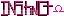-=+=- -=+=- -=+=- -=+=- -=+=- -=+=- -=+=- -=+=- -=+=- -=+=- -=+=- -=+=- -=+=- -=+=- -=+=- -=+=- -=+=- -=+=- -=+=- -=+=- -=+=- -=+=- -=+=- -=+=- -=+=- -=+=- -=+=- -=+=- -=+=- -=+=- (c) WidthPadding Industries 1987 0|74|0 -=+=- -=+=- -=+=- -=+=- -=+=- -=+=- -=+=- -=+=- -=+=- -=+=- -=+=- -=+=- -=+=- -=+=- -=+=- -=+=- -=+=- -=+=- -=+=- -=+=- -=+=- -=+=- -=+=- -=+=- -=+=- -=+=- -=+=- -=+=- -=+=- -=+=-
SoCoder -> Snippet Home -> Variables

HoboBenCreated : 22 April 2011
Edited : 25 April 2011
Language : Monkey

### Circular Buffer (C)

A fast fixed-size buffer. which you can pretend is "infinite memory" for data that you don't care too much about.

When you write to it, it fills up until you start overwriting the oldest data. When reading, it returns the oldest data it has.

Ideal for buffering data anywhere a bandwidth is a problem, like text input when you have a low framerate, or streaming real-time sound or video where the past doesn't matter too much.

It looks simple, but was a helluva thing to implement as the intuitive solutions (like checking if start == end for detecting emptiness) don't work. So hopefully it saves somebody else the trouble!

If you like syntax highlighting, syntax highlighted version (needs JavaScript)

Monday, 25 April 2011, 14:09
SchererererBUFFER_SIZE isn't defined in this snippet, shouldn't it be defined so that it can be used, so that we can change this:

to this:Monday, 25 April 2011, 14:50
HoboBenWhoops! Yes, you're right. Thanks for the correction.